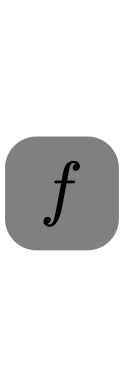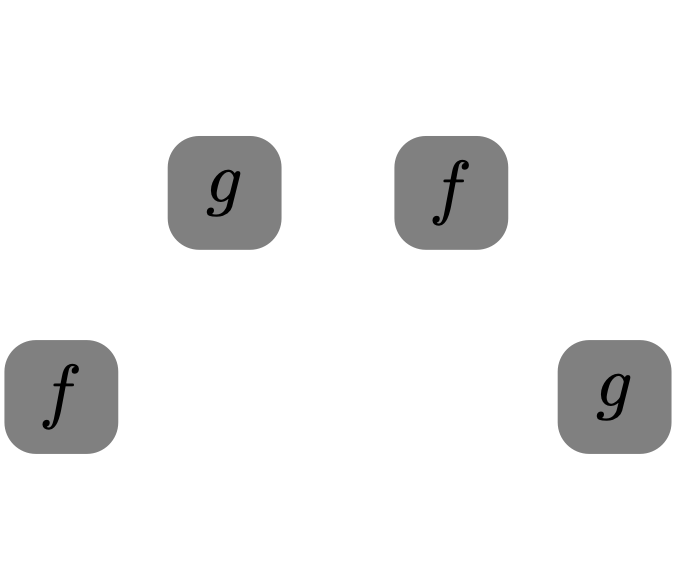## Research

I study fusion categories using graphical calculus and operator algebraic techniques.

### What are fusion categories?

A fusion category is a type of monoidal category, which is a category with higher structure. Fusion categories are best understood by looking at examples. The simplest example of a fusion category is $$\mathsf{Vec}_{\operatorname{fd}}$$, the category of finite-dimensional (complex) vector spaces equipped with the standard tensor product. Here, the tensor product endows $$\mathsf{Vec}_{\operatorname{fd}}$$ with the structure of a fusion category. Note that for all vector spaces $$V \in \mathsf{Vec}_{\operatorname{fd}}$$, we have that $$\mathbb{C} \otimes V \cong V \otimes \mathbb{C} \cong V$$. Thus, the vector space $$\mathbb{C}$$ acts as the "tensor identity," which is called the tensor unit. Also, note that every finite-dimensional vector space is isomorphic to the finite direct sum of copies of the tensor unit $$\mathbb{C}$$. As a result, $$\mathsf{Vec}_{\operatorname{fd}}$$ is the trivial fusion category.

We can get more interesting examples of fusion categories as follows. Let $$G$$ be a finite group. We can then consider the category $$\mathsf{Vec}_{\operatorname{fd}}(G)$$ of finite-dimensional $$G$$-graded vector spaces. Note that $$G$$-graded vector spaces are vector spaces $$V$$ of the form $$V \cong \bigoplus_{g \in G} V_g$$, and maps between $$G$$-graded vector spaces must respect the grading. The tensor product structure on $$\mathsf{Vec}_{\operatorname{fd}}(G)$$ is given by $$\mathbb{C}_g \otimes \mathbb{C}_h \cong \mathbb{C}_{gh}$$ for $$g, h \in G$$. In this case, $$\mathbb{C}_1$$ is the tensor unit, and every vector space $$V \in \mathsf{Vec}_{\operatorname{fd}}(G)$$ is isomorphic to the direct sum of vector spaces of the form $$\mathbb{C}_g$$ for $$g \in G$$. The vector spaces $$\mathbb{C}_g$$ for $$g \in G$$ are representatives for the isomorphism classes of what are called simple objects in the category $$\mathsf{Vec}_{\operatorname{fd}}(G)$$. Another example of a fusion category is $$\mathsf{Fib}$$, which has two simple objects $$1$$ and $$\tau$$ (up to isomorphism). In this category, $$1$$ is the tensor unit, and $$\tau^2 \cong 1 \oplus \tau.$$

We can now summarize the salient features of a fusion category: a fusion category is a linear category (hom sets are finite-dimensional vector spaces) with a tensor product structure, where every object can be written as the direct sum of simple objects and the tensor unit is also a simple object. In the example $$\mathsf{Vec}_{\operatorname{fd}}(G)$$, every simple object is invertible, as for each $$g \in G$$, we have that $$\mathbb{C}_g \otimes \mathbb{C}_{g^{-1}} \cong \mathbb{C}_{g^{-1}} \otimes \mathbb{C}_{g} \cong \mathbb{C}_1$$. Not every fusion category needs to satisfy this property, and in fact, $$\mathsf{Fib}$$ is the simplest one that does not.

### What is the graphical calculus?

The graphical calculus is a way of representing fusion categories (and more generally, monoidal categories) visually, where strings are used to represent objects, coupons are used to denote morphisms, vertical stacking is used for composition of morphisms, and horizontal juxtaposition denotes the tensor product. For example, the following diagram represents a map $$f \colon a \to b \otimes c$$.(Note that we read diagrams from bottom to top, although some use different conventions.) Applying planar isotopy to these diagrams preserves equality. For instance, we have the following equality, which is called the exchange relation.Algebraically, the exchange relation is written $(\operatorname{id}_b \otimes g) \circ (f \otimes \operatorname{id}_c) = (f \otimes \operatorname{id}_d) \circ (\operatorname{id}_a \otimes g).$ While the exchange relation can be easily verified algebraically, more complicated equalities are often proven much more easily with the graphical calculus, and hence it can be a very powerful tool.

## Contact

Email: wallick (dot) 43 (at) osu (dot) edu
Office: Math Tower 200## Elements

### Text

This is bold and this is strong. This is italic and this is emphasized. This is superscript text and this is subscript text. This is underlined and this is code: for (;;) { ... }. Finally, this is a link.

#### Blockquote

Fringilla nisl. Donec accumsan interdum nisi, quis tincidunt felis sagittis eget tempus euismod. Vestibulum ante ipsum primis in faucibus vestibulum. Blandit adipiscing eu felis iaculis volutpat ac adipiscing accumsan faucibus. Vestibulum ante ipsum primis in faucibus lorem ipsum dolor sit amet nullam adipiscing eu felis.

#### Preformatted

i = 0;

while (!deck.isInOrder()) {
print 'Iteration ' + i;
deck.shuffle();
i++;
}

print 'It took ' + i + ' iterations to sort the deck.';

### Lists

#### Unordered

• Dolor pulvinar etiam.
• Felis enim feugiat.

#### Alternate

• Dolor pulvinar etiam.
• Felis enim feugiat.

#### Ordered

1. Dolor pulvinar etiam.
2. Etiam vel felis viverra.
3. Felis enim feugiat.
4. Dolor pulvinar etiam.
5. Etiam vel felis lorem.
6. Felis enim et feugiat.

### Table

#### Default

Name Description Price
Item One Ante turpis integer aliquet porttitor. 29.99
Item Two Vis ac commodo adipiscing arcu aliquet. 19.99
Item Three Morbi faucibus arcu accumsan lorem. 29.99
Item Four Vitae integer tempus condimentum. 19.99
Item Five Ante turpis integer aliquet porttitor. 29.99
100.00

#### Alternate

Name Description Price
Item One Ante turpis integer aliquet porttitor. 29.99
Item Two Vis ac commodo adipiscing arcu aliquet. 19.99
Item Three Morbi faucibus arcu accumsan lorem. 29.99
Item Four Vitae integer tempus condimentum. 19.99
Item Five Ante turpis integer aliquet porttitor. 29.99
100.00